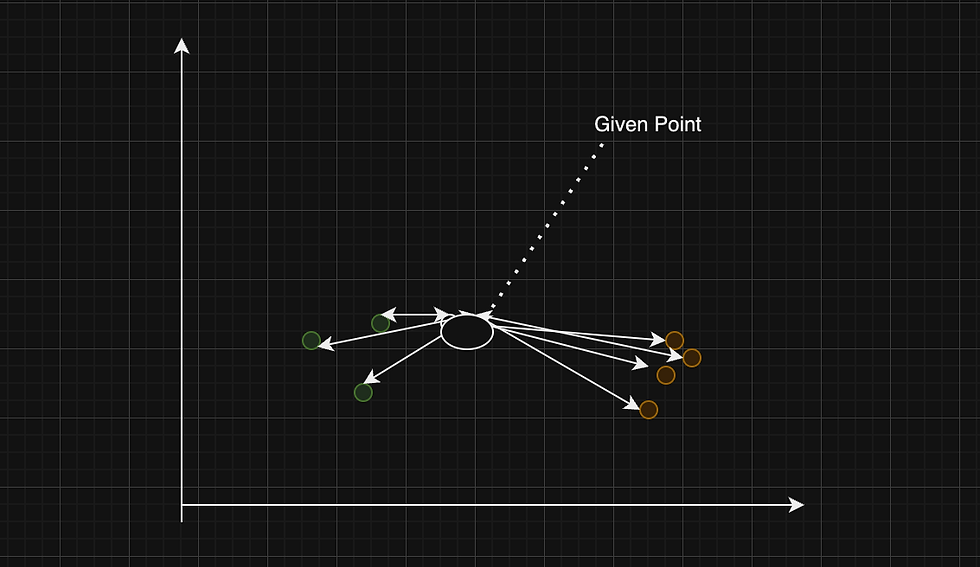top of pageSearch

# SVM and KNN algorithms :

Support Vector Machine(SVM) :

Support Vector Machine(SVM) comes under Supervised learning algorithm which can be used for both regression, classification in machine learning. Support vector machine can also be used for outliers detection. Applications that vastly uses support vector machine are for face detection, classification of images, text categorization etc.,

To understand SVM thoroughly we should know that the categories are divided by a hyperplane along with two margins. These margins are drawn parallel to hyperplane with respect to the nearest values of the categories that are present. The straight line represents the hyperplane and the dotted lines are also hyperplanes which represent as the margin lines.The model which contains the largest margin distance will be considered as the best svm to work. The points that are passing through the marginal hyperplane is called as the support vectors. Linear Seperable can be handled by svm hyperplanes, but non linear Seperable should use kernel svm. svm kernels helps us to convert low dimensional to high dimensional so that hyperplanes can be created.(eg., 2dimensional can be converted into 3dimensional or 1-dimensional can be converted into 2-dimensional).

SVM can be mostly used for sample datasets or small datasets, as it does not perform well for large datasets. SVM can handle both linear and non linear datasets by changing its dimensionality to higher dimensionality. As the no.of features increases the performance of the SVM decreases.

Kernel trick helps us to analyze and find patterns in the non-linearly separable dataset , kernel helps in adding the dimension to the non linear dataset (2d to 3d) and to create a hyperplane for the new dimension of the dataset. SVM also helps in predicting the output very quickly with good accuracy. SVM also tries to reduce the distance between the margins(The distance between the support vectors).

K Nearest Neighbour (KNN) :

K Nearest neighbour (KNN) is a Supervised algorithm which can be used for both regression and classification in machine learning. Applications KNN algorithm are audio detection and video detection. KNN imputation is vividly used in data preprocessing or to handle missing values in data. As the data do not allow any null or missing before using machine learning many features can be replaced by using KNN imputation. In the below diagram we can observe the KNN algorithm implemented on the data which contains two categories of data. The value of K is used to find the performance tuning and it is used for finding best accuracy. In simple terms the no.of neighbors we are considering is the value of K. The distance can be calculated by using parameters eucledian and manhattan.From the given key point to other keys the distance will be calculated and it will be saved as the low K values with low bias and high variance overfitting, high K values with high bias and low variance underfitting. Whereas the good K value have the balance between overfitting and underfitting.

While implementing KNN algorithm the K value should be calculated and then the mean of all the nearesr=t neighbors should be calculated to find the regression of the KNN. While implementing KNN classifier K value should be calculated and no.of neighbor points are nearest to the given point should be considered, the category which have maximum no.of nearest neighbors will be considered as the given point.

Both SVM and KNN algorithms plays a major role in Supervised learning, both the algorithms works effectively on small datasets compared to large datasets. But they give effective results for the small datasets.